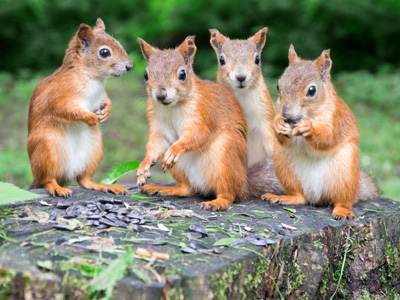How many squirrels? 1 + 3 = 4 in total.

# Year 1 Calculation - Addition and Total

This quiz addresses the requirements of the National Curriculum KS1 Maths and Numeracy for children aged 5 and 6 in year 1. Specifically this quiz is aimed at the section dealing with finding the total.

Understanding and using the words associated with addition is an important first step when calculating. Recognising that 'finding the total' means finding how much or how many there are altogether, especially when groups of numbers or objects are added, is important as 'total' is one of the first addition words Year 1 children will encounter.

To see a larger image, click on the picture.
Question 1
How many chocolates in total?
12
14
15
16
4 + 4 + 4 + 4 gives a total of 16
Question 2
How many dogs in total?
5
6
7
8
There is a total of 5 dogs here
Question 3
How many fish are there in total?
15
12
9
10
There is a total of 10 fish. Did you spot them all?
Question 4
What is the total number of crayons in this pot?
11
12
10
13
There is a total of 12 crayons
Question 5
What is the total number of birds?
9
8
10
7
2 + 5 + 2 gives a total of 9
Question 6
How many stars in total?
8
4
6
9
4 + 4 gives a total of 8
Question 7
What does 'total' mean?
A subtraction must be done
How many there are altogether
What you get when you divide the numbers
What you get if you count backwards
The total is how many there are altogether if you count or add everything together
Question 8
How many socks in total?
6
2
4
8
4 pairs of socks is a total of 8
Question 9
How many children in total?
8
9
7
10
There are 10 children in total
Question 10
What is the total shown on the two dice?
3
4
7
10
4 + 3 gives a total of 7